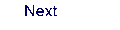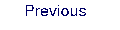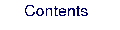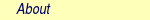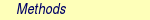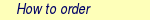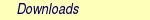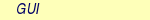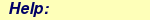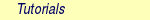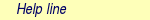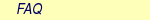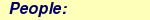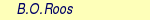MOLCAS manual:Next: 8.2 averd Up: 8. Programs Previous: 8. Programs

Subsections

This module is automatically invoked by the Slapaf module. This is the preferred mode of operation! In connection with numerical gradients it will ensure that the rotational and translational invariance is fully utilized in order to reduce the number of used displacements.

The Alaska module compute analytic or for numerical gradients requests the execution of an alternative module. The Alaska module figures out the method automatically. Analytic methods are implemented for the HF, MBPT2, KS-DFT, and RASSCF and SA-CASSCF method. Numerical methods are implemented for SCF, KS-DFT, RASSCF, MBPT2, CCSDT, the CASPT2 and MS-CASPT2 methods, including the use of the Cholesky decomposition for the methods were that has been implemented.

Both analytic and numerical procedures are parallelized.

For SA-CASSCF gradient the Alaska module will automatically start up the MCLR module if required.

Gradients of the energy with respect to nuclear coordinates can be computed for any type of wave function as long as an effective first order density matrix, an effective Fock matrix, and an effective second order density matrix is provided. The term effective is related to that these matrices in the case of non-variational parameters in the wave function (e.q. CI, MP2, CASPT2, etc.) are modified to include contributions from the associated Lagrange multipliers. The gradient expression apart from these modifications is the same for any wave function type. ALASKA is the gradient program, which will generate the necessary integral derivatives and combine them with the matrices mentioned in the text above.

## 8.1.2 Description

ALASKA is written such that gradients can be computed for any kind of basis function that SEWARD will accept.

ALASKA is able to compute the following integral derivatives:

• overlap integrals,
• kinetic energy integrals,
• nuclear attraction integrals (point charges or finite nuclei),
• electron repulsion integrals,
• external electric field integrals,
• ECP and PP integrals,
• reaction field integrals,
• and Pauli repulsion integrals.

ALASKA employs two different integration schemes to generate the one- and two-electron integral derivatives. The nuclear attraction and electron repulsion integrals are evaluated by a modified Rys-Gauss quadrature . All other integral derivatives are evaluated with the Hermite-Gauss quadrature. The same restriction of the basis sets applies as to SEWARD. None of the integral derivatives are written to disk but rather combined immediately with the corresponding matrix from the wave function.

At present the following limitations are built into ALASKA:

 Max number of unique basis functions: 2000 Max number of symmetry independent centers: 500 Highest angular momentum: 15 Highest symmetry point group: D2h

The module is parallelized over the displacements, which in case of large jobs gives a linear speed up compared to a serial execution, although in order to obtain this it is important to choose the number of nodes such that the number of contributing perturbations is a multiple of the number of nodes. For a given molecule the number of perturbations equals the number of atoms times 6 (a perturbation with plus and minus delta for each of the three axes). Symmetry can of course reduce this number.

## 8.1.4 Dependencies

ALASKA depends on the density and Fock matrices generated by SCF or RASSCF. In addition it needs the basis set specification defined in SEWARD. The dependencies of the numerical part of the module is the union of the dependencies of the SEWARD, SCF, RASSCF, MBPT2, MOTRA, CCSDT, and CASPT2 modules. All these dependencies, however, are totally transparent to the user.

## 8.1.5 Files

### 8.1.5.1 Input files

Apart from the standard input unit ALASKA will use the following input files: RYSRW, ABDATA, ONEINT, RUNFILE (for more information see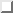).

The files of the SEWARD, SCF, RASSCF, MBPT2, MOTRA, CCSDT, and CASPT2 modules are needed for the numerical procedure.

### 8.1.5.2 Output files

In addition to the standard output unit ALASKA will generate the following files.

 File Contents RUNFILE The runfile is updated with information needed by the SLAPAF relaxation program. ALASKA will write the molecular Cartesian gradients on this file. ALASKA.INPUT File with the latest input processed by ALASKA.

## 8.1.6 Input

Below follows a description of the input to ALASKA. Note that input options are related to the analytic gradient procedure if not otherwise noted!

In addition to the keywords and the comment lines the input may contain blank lines. The input is always preceded by the program name:

 Keyword Meaning TEST With this keyword the program will process only the input. It is a debugging aid to help you check your input. NAC Requests a calculation of the nonadiabatic coupling vector between the two specified roots in a SA-CASSCF calculation. NOCSF In a NAC calculation, neglects the so-called CSF contribution. Note that this contribution is responsible for the translational and rotational non-invariance, and it has been suggested that not including it may give more physical results in dynamics simulations . ONEOnly Compute only the nuclear repulsion and one-electron integrals contribution to the gradient. The default is to compute all contributions to the molecular gradient. CUTOff Threshold for ignoring contributions to the molecular gradient follows on the next line. The default is 1.0d-7. The prescreening is based on the 2nd order density matrix and the radial overlap contribution to the integral derivatives. OFEMbedding Performs a Orbital-Free Embedding gradient calculation, available only in combination with Cholesky or RI integral representation. The runfile of the environment subsystem renamed AUXRFIL is required. An example of input for the keyword OFEM is the following: OFEMbedding   ldtf/pbe dFMD   1.0 (see the OPTIONAL keyword :kword:`DFMD` below). The keyword OFEM requires the specification of two functionals in the form fun1/fun2, where fun1 is the functional used for the Kinetic Energy (available functionals: Thomas-Fermi, with acronym LDTF, and the NDSD functional), and where fun2 is the xc-functional (LDA, LDA5, PBE and BLYP available at the moment). DFMD In combination with OFEM, specifies the fraction of correlation potential to be added to the OFE potential (zero for KSDFT and one for HF). POLD The gradient is printed in the old format. Note: by default gradient is not printed any longer. PNEW The gradient is printed in the new human-readable format. VERBose The output will be a bit more verbose. SHOW gradient contributions The gradient contributions will be printed.